3D Rotations in Processing (Vectors, Matrices, Quaternions)A 3D shape easing between rotations.

Creating A Vector from Azimuth and Inclination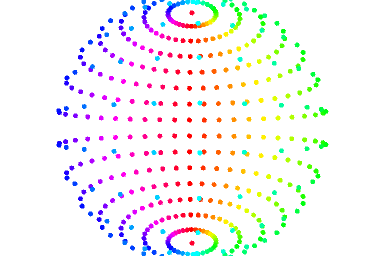A sphere constructed with fromAngle.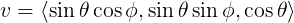v = < sine inclination cosine azimuth, sine inclination sine azimuth, cosine inclination >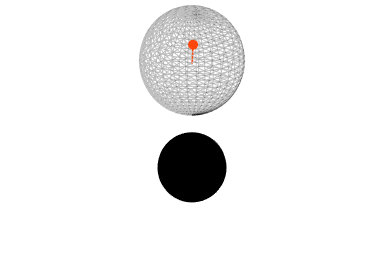A directional light calculated from azimuth and inclination.

Rotations of a Vector by Axis and Angle

Project The Point Onto The Axis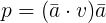The projection of point v equals the dot product of v and axis a multiplied by a.

Find the Elevation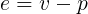The elevation of point v equals v minus its projection onto axis a.

Find the Plane of Rotation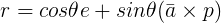The plane of rotation r equals cosine theta multiplied by the elevation plus sine theta multiplied by the axis a crossed with projection p.

Extend a Rotated Point to the Original’s Plane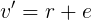The rotation of v around axis a equals the rotation r plus the elevation.

4 x 4 Matrices

Looking Toward A Point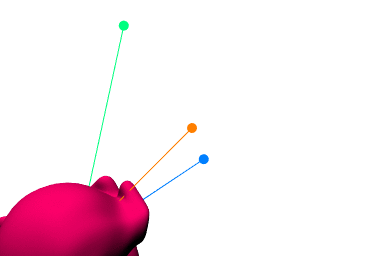Orienting a model to look at a target point.

Quaternions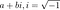A complex number is a real part, a, added to an imaginary part, b.Hamilton’s discovery.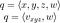A quaternion can be described with four components, x, y, z and w; or as an imaginary vector v and a real part w.

Converting from Axis-Angles, Applying To Points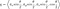A quaternion’s imaginary parts are the normalized axis a multiplied by sine half theta. Its real part is cosine half theta.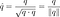A unit quaternion is normalized by dividing the quaternion by its magnitude, or the square-root of its dot product with itself.

Easing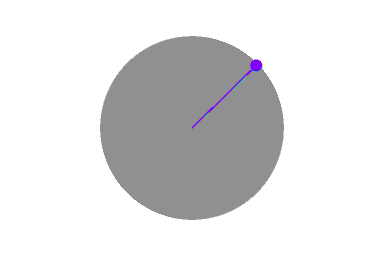Linear interpolation, normalized linear interpolation and spherical interpolation.

Converting To and From Matrices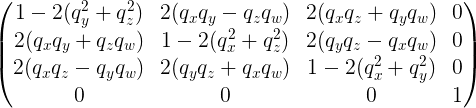Conversion from a quaternion to a matrix.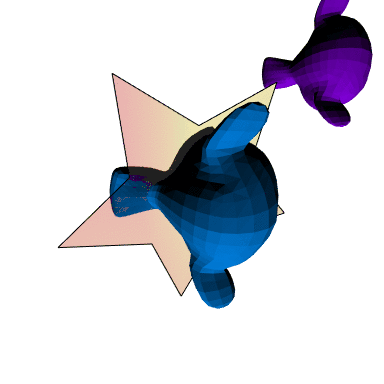A quaternion rotation applied to an immediate shape (star), retained shape (blue Suzanne), tested against a control shape (purple Suzanne).

Conclusion

Welcome to a place where words matter. On Medium, smart voices and original ideas take center stage - with no ads in sight. Watch
Follow all the topics you care about, and we’ll deliver the best stories for you to your homepage and inbox. Explore
Get unlimited access to the best stories on Medium — and support writers while you’re at it. Just \$5/month. Upgrade